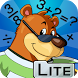# Math Trek K-3 LiteFree App

Rated 3.00/5 (6) —  Free Android application by NECTAR

## About Math Trek K-3 Lite

Math Trek K-3 Lite is a sampler of 6 engaging math activities supporting common-core learning for students in kindergarten to Grade 3. Students explore and learn mathematical concepts in creative settings, such as a farm, a ship, a grocery store, and a toy store. A friendly bear, named after the famous mathematician Fibonacci, serves as a guide for this easy to use app.
·Data Analysis, Math Trek K: Farm Mixup
o Sort and graph farm animals using a bar chart.
·Number & Operations, Math Trek 1: Ship's Ahoy
o Use the numbers on a sail boat to create addition and subtraction equations.
·Geometry Math Trek 1: Shopping for Shapes
o Match common items from the grocery store with drawings of three-dimensional figures (e.g. cube, cone, cylinder, sphere).
·Algebra, Math Trek 2: Bouncing Buttons
o Sort buttons using two attributes such as color and shape.
·Measurement, Math Trek 3: Everyday Temperatures (Metric)
o Make measurements such as reading a thermometer and recording the temperature for everyday situations.
·Measurement, Math Trek 3: Picture This (Imperial)
o Make measurements such as reading a thermometer and recording the temperature for everyday situations.

Watch for these and many more activities for K-3, coming soon in apps from NECTAR Learning:
Math Trek K (22 activities)
Math Trek 1 (50 activities)
Math Trek 2 (47 activities)
Math Trek 3 (30 activities)
and for a review of the fundamentals for students in Grades 4 and up:
Math Trek Geometry (25 activities)
Math Trek Algebra (16 activities)
Math Trek Number and Operations (53 activities)
Math Trek Measurement (35 activities)
Math Trek Data Analysis (20 activities)

Free
downl.
Android
2.2+
n/a
Not
rated
Android app

S70%
by S####:

Rahul kumar

RATING
3.05
6 users

5

4

3

2

1